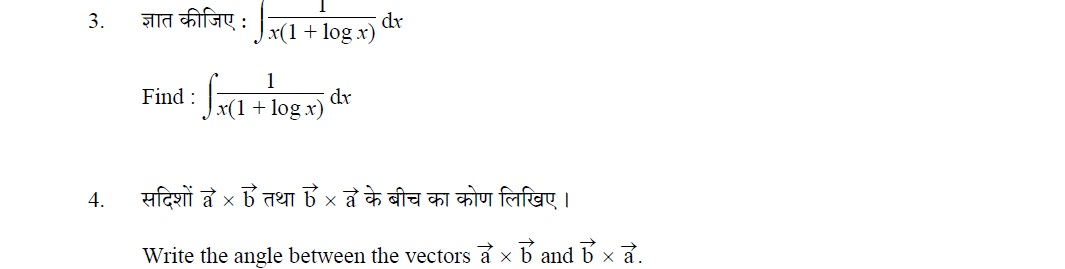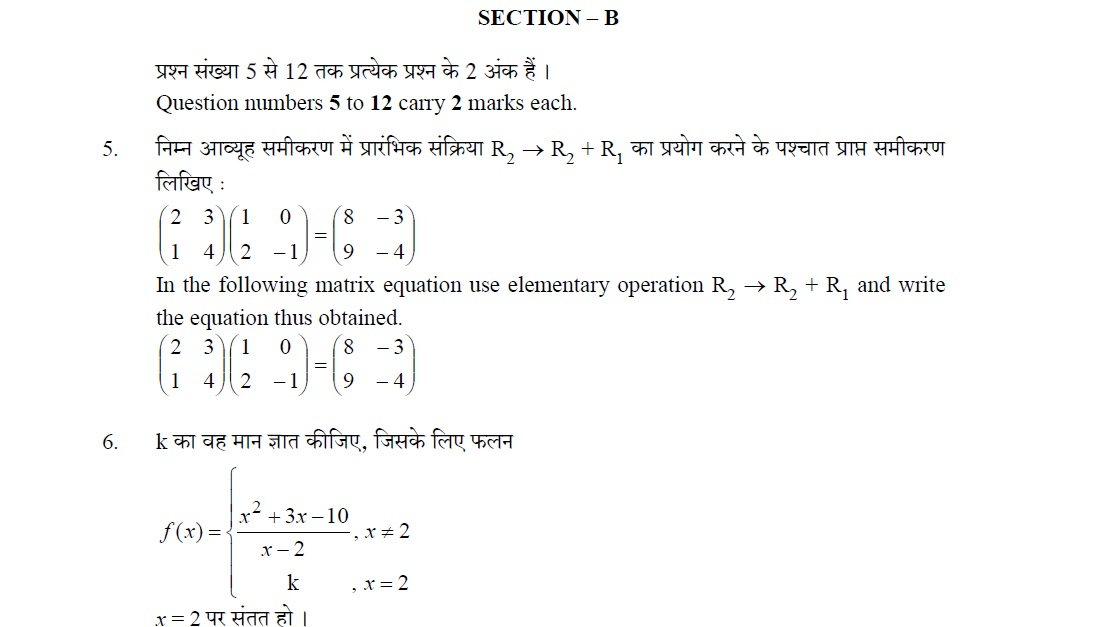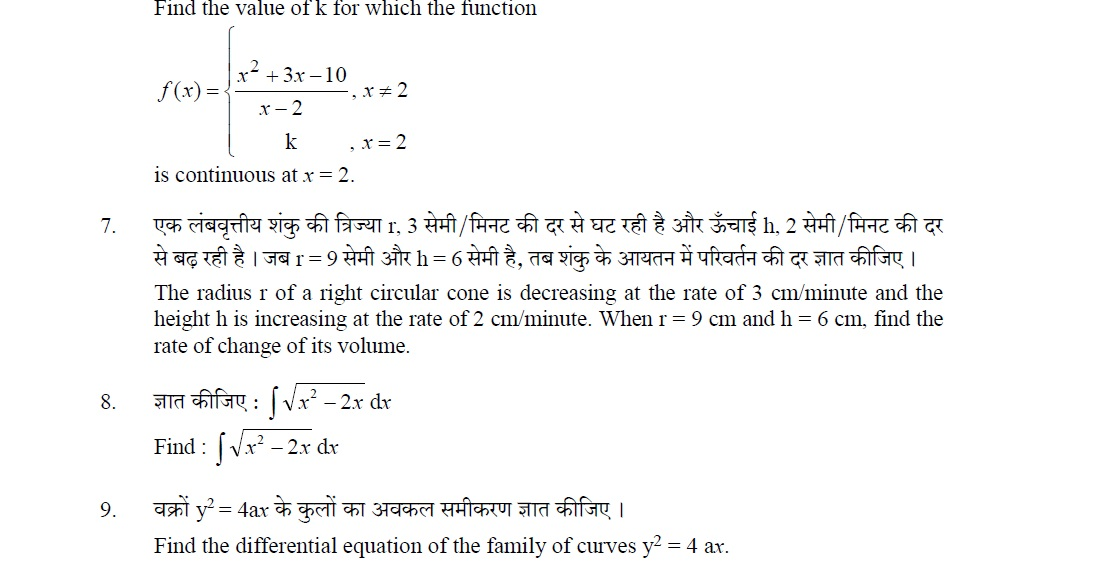# CBSE Class-12 Question Papers for IOP/Comptt Examination 2017 : Delhi Scheme, Mathematics

Disclaimer: This website is NOT associated with CBSE, for official website of CBSE visit - www.cbse.gov.in## CBSE Class-12 Question Papers for IOP/Comptt Examination 2017 : Mathematics, Set -1

MATHEMATICS

Time allowed : 3 hours

Maximum Marks : 100

General Instructions :
(i) All questions are compulsory.
(ii) This question paper consists of 29 questions divided into four sections A, B, C and D. Section A comprises of 4 questions of one mark each, Section B comprises of 8 questions of two marks each, Section C comprises of 11 questions of four marks each and Section D comprises of 6 questions of six marks each.
(iii) All questions in Section A are to be answered in one word, one sentence or as per the exact requirement of the question.
(iv) There is no overall choice. However, internal choice has been provided in 3 questions of four marks each and 3 questions of six marks each. You have to attempt only one of the alternatives in all such questions.
(v) Use of calculators is not permitted. You may ask for logarithmic tables, if required.

SECTION – A

Question numbers 1 to 4 carry 1 mark each.
1. Let A and B are matrices of order 3 x 2 and 2 x 4 respectively. Write the order of matrix (AB).

2. Write the equation of tangent drawn to the curve y = sin x at the point (0, 0).